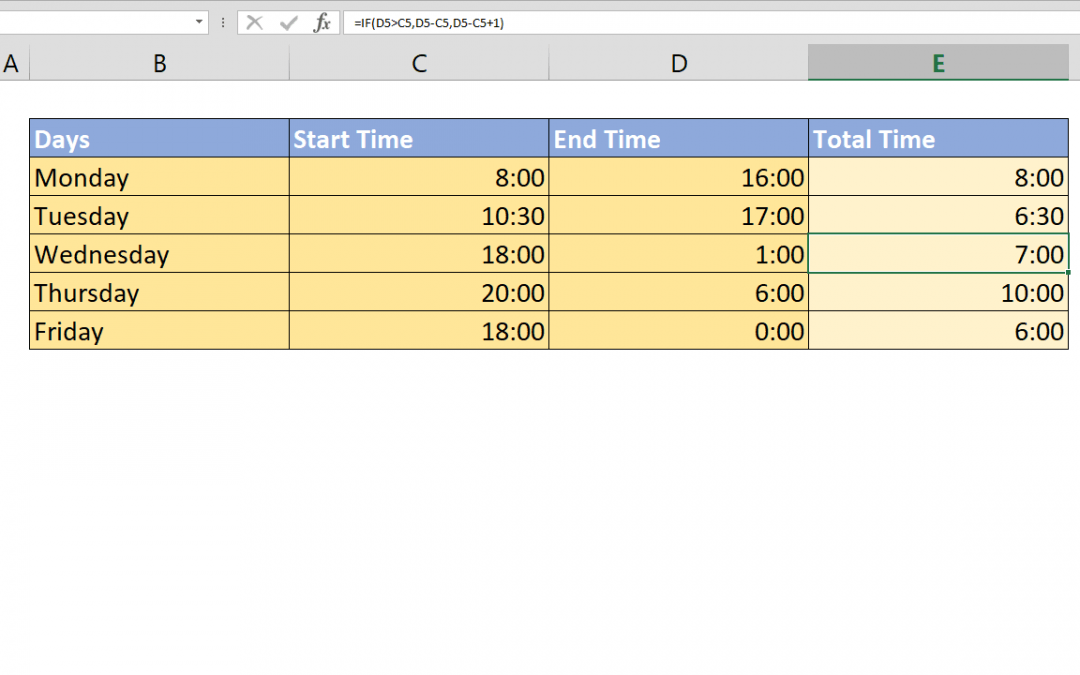## How to use IFS and SWITCH formulas instead of nested IF statements

Nested IF statements are useful for creating more complicated scenarios in Excel. However, IFS and SWITCH formulas can work more efficiently when dealing with more than one condition. These formulas work a bit differently than the legacy IF function. Let’s start...## How to create a time calculator in Excel

When you need to calculate the difference between a time present day and the next day, calculations can get messed up and you will see lots of # characters. This article shows how to create a time calculator in Excel that can calculate shift time after midnight using...## How to create conditional Excel drop down lists

It’s often a good idea to use drop down lists or combo boxes to limit user errors make the data more standardized. However, sometimes we want our drop downs to populate under some conditions. Here, you can find out how to create conditional Excel drop down using Data...## How to lookup across two tables with VLOOKUP and IF functions

How to lookup across two tables by a condition is a combination of the VLOOKUP and the IF functions. The IF function handles which table and VLOOKUP handles what to search for. Syntax =VLOOKUP(lookup value, IF(condition, first table if condition is TRUE, second table...## How to find smallest value in array using a criteria

This article shows how to find smallest value in array using SMALL and IF functions in an array formula. Syntax {=SMALL(IF(criteria range = criteria, data range reference), nth)} Steps Start with =SMALL( function Continue with IF( Type the criteria range-criteria...## How to find largest value in array using a criteria

This article shows how to find largest value in array using LARGE and IF functions in an array formula. Syntax {=LARGE(IF(criteria range = criteria, data range reference), nth)} Steps Start with =LARGE( function Continue with IF( to apply condition Type the criteria...